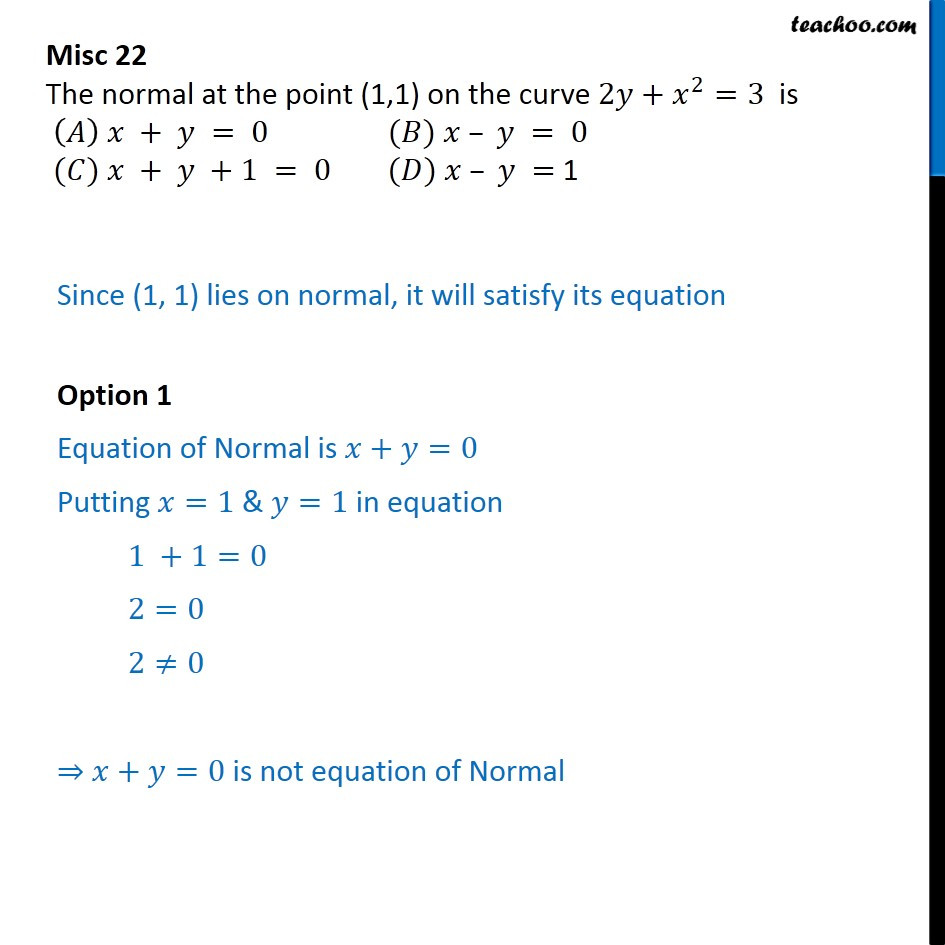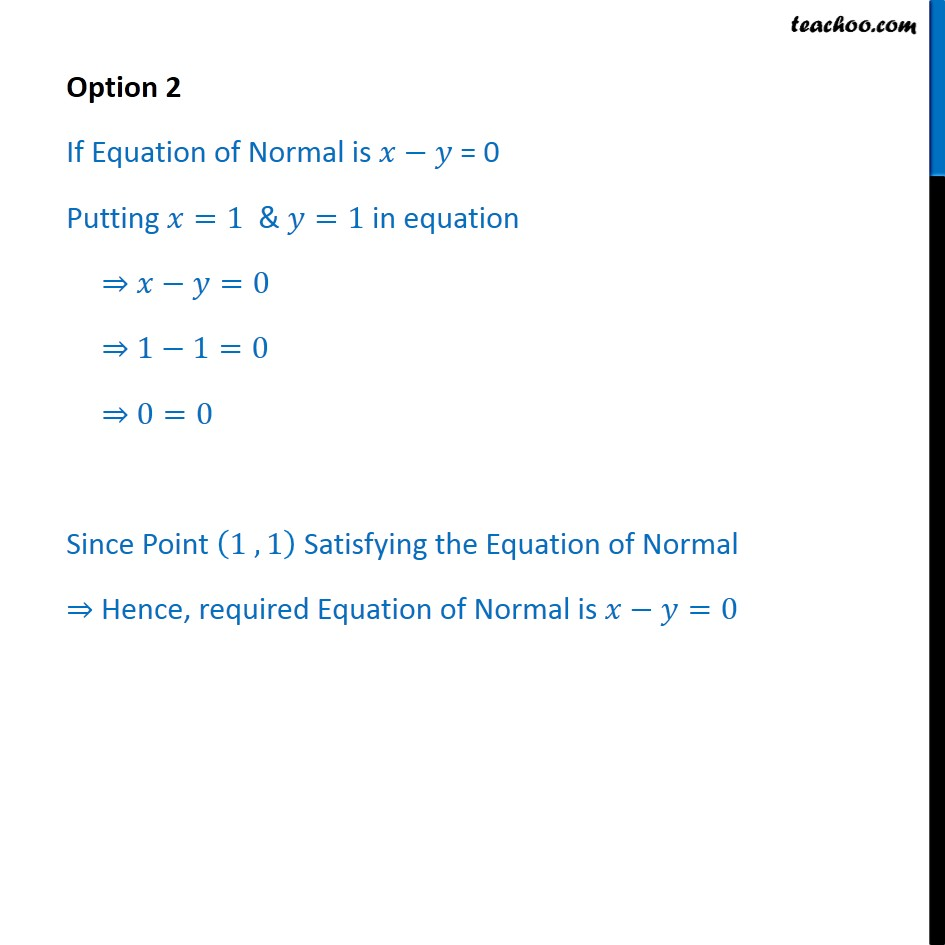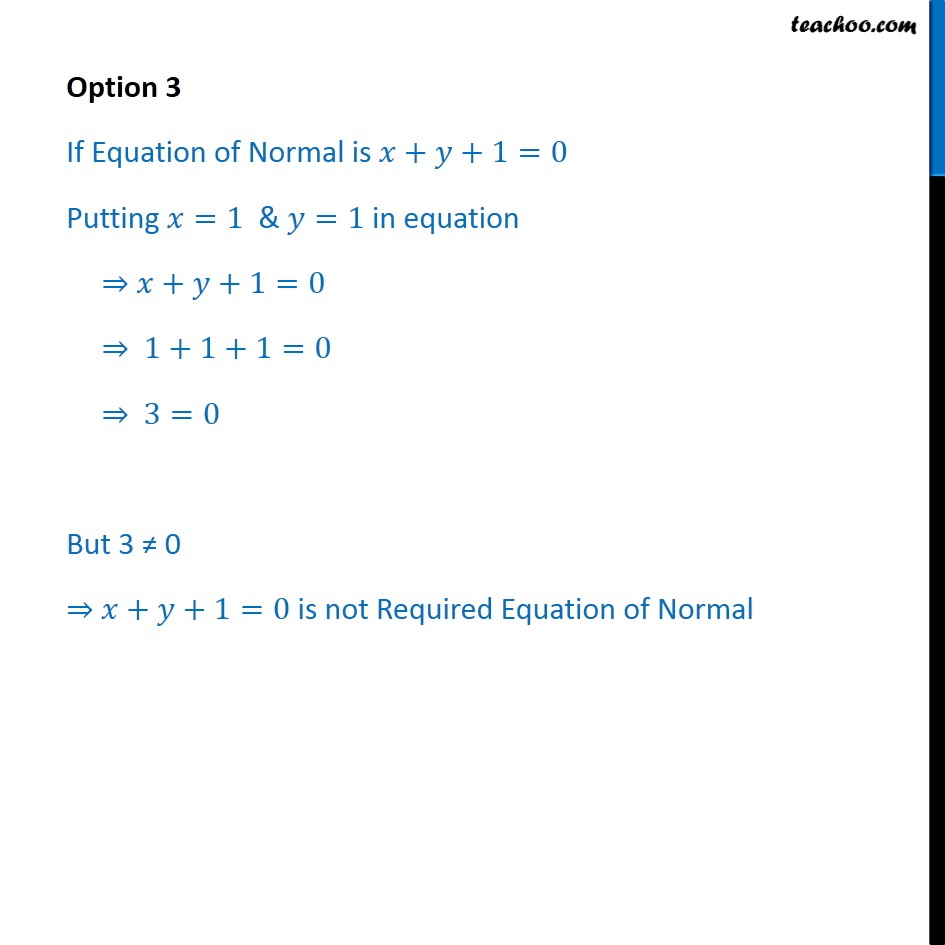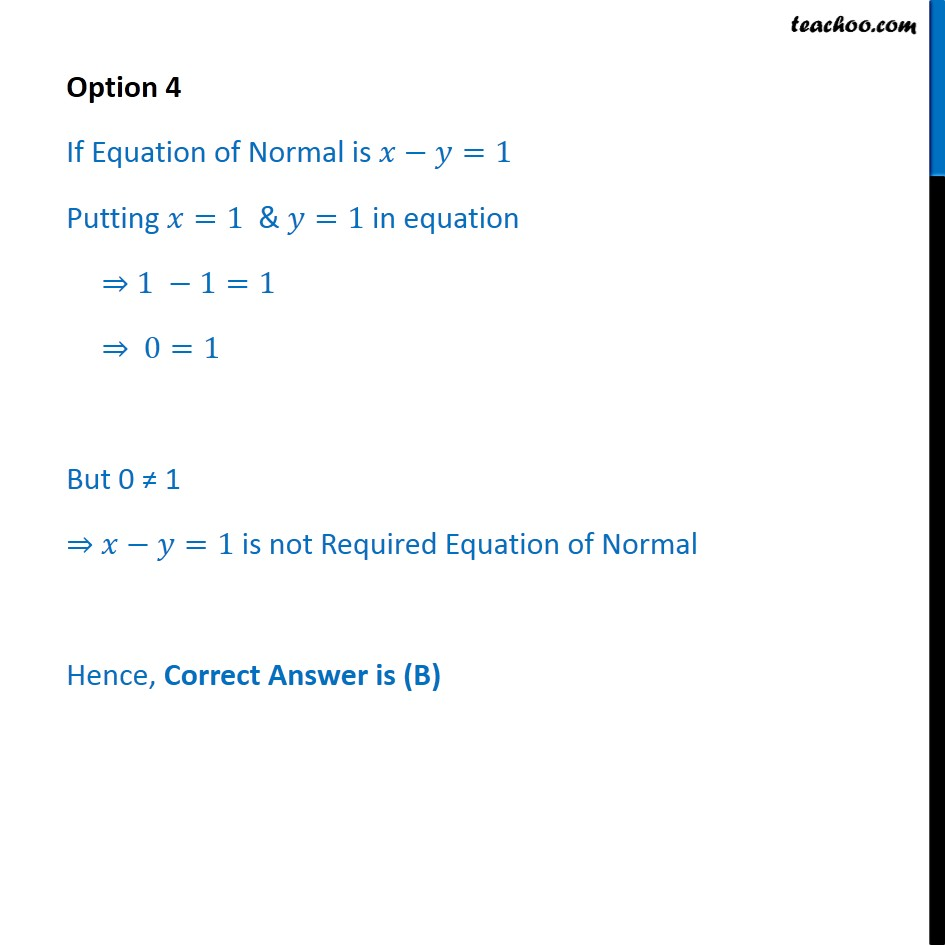Subscribe to our Youtube Channel - https://you.tube/teachoo

1. Chapter 6 Class 12 Application of Derivatives
2. Serial order wise
3. Miscellaneous

Transcript

Misc 22 The normal at the point (1,1) on the curve 2 + 2 =3 is + = 0 ( ) = 0 ( ) + +1 = 0 ( ) = 1 Since (1, 1) lies on normal, it will satisfy its equation Option 1 Equation of Normal is + =0 Putting =1 & =1 in equation 1 +1=0 2=0 2 0 + =0 is not equation of Normal Option 2 If Equation of Normal is = 0 Putting =1 & =1 in equation =0 1 1=0 0=0 Since Point 1 , 1 Satisfying the Equation of Normal Hence, required Equation of Normal is =0 Option 3 If Equation of Normal is + +1=0 Putting =1 & =1 in equation + +1=0 1+1+1=0 3=0 But 3 0 + +1=0 is not Required Equation of Normal Option 4 If Equation of Normal is =1 Putting =1 & =1 in equation 1 1=1 0=1 But 0 1 =1 is not Required Equation of Normal Hence, Correct Answer is (B)

Miscellaneous